Length parameters of cotton fibers | Textile Study Center

Length parameters of cotton fibers

7.88K
0Md Sohanur Rahman Sobuj

Co-founder , Admin & Author at Textile Study Center
Student of Bangladesh University of Textiles (BUTEX) Department: Apparel Engineering+Md Sohanur Rahman SobujLength parameters of cotton fibers

Definition of length is based on two criteria- one based on its ‘full length’ i.e. end to end lengths (staple length, ML, EL, UHML,UQL) and the other based on ‘span length’(2.5% span length,50% span length, ML & UHML).

Mean length:

It is the arithmetic mean of the length of all the fibers present in a sample of the cotton. It can be calculated by number or weight of fibers.

Let us consider three fibers length (mm) and weight are  l1,l2,land w1,w2,wrespectively.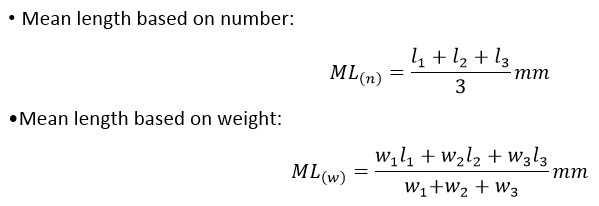Upper Half Mean Length (UHML):

UHML is the mean length by the number of fibers in the largest half by weight of fibers in a cotton sample, usually measured from the fibrogram. Upper half mean length is normally equivalent to the staple length.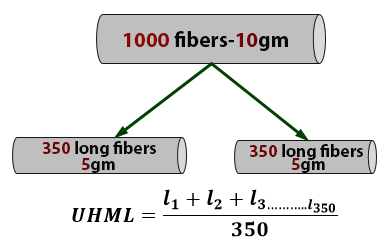Uniformity Index (UI):

The ratio between mean length (ML) & Upper half quartile length is called uniformity index, express as a percentage.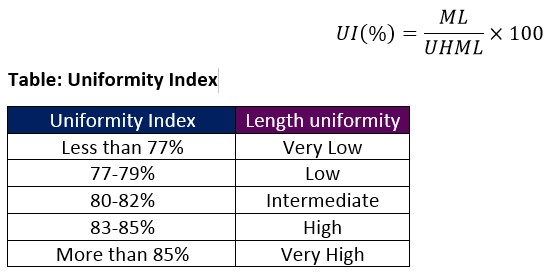Staple length:

The most frequent length in a fibrous sample is called staple length. Staple length is one of the most important factors of cotton quality because both ﬁber ﬁneness and fiber tensile strength are associated with staple length. The longer staples are usually finer and stronger than the shorter staples.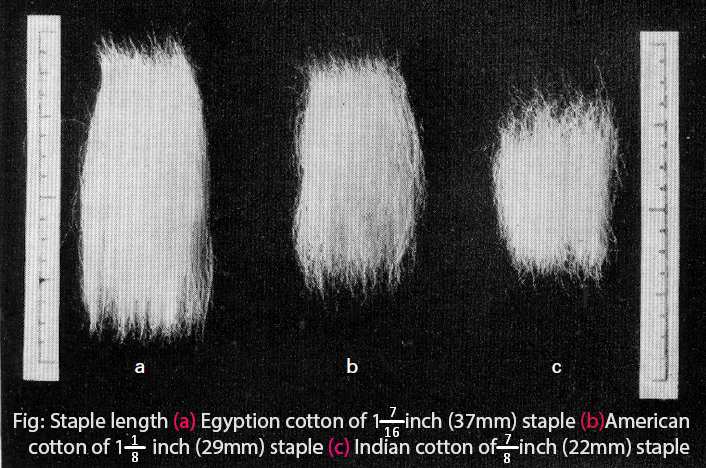Staple length can be of following types: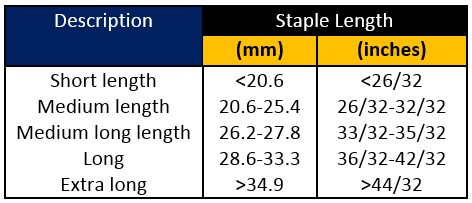Staple diagram:

Graphical representation of the end aligned fiber beard (arrangement of fibers by the length in decreasing order starting from a horizontal line) is called staple diagram.

Cumulative frequency fiber length distribution (CFD) is also called the staple diagram (in short staple spinning) or diagram of Hauteur (worsted spinning). It is produced by sorting, either by number or by weight, the straightened lengths of the individual ﬁbers making the sample. Generally, the CFD is obtained manually by the Suter-Webb method.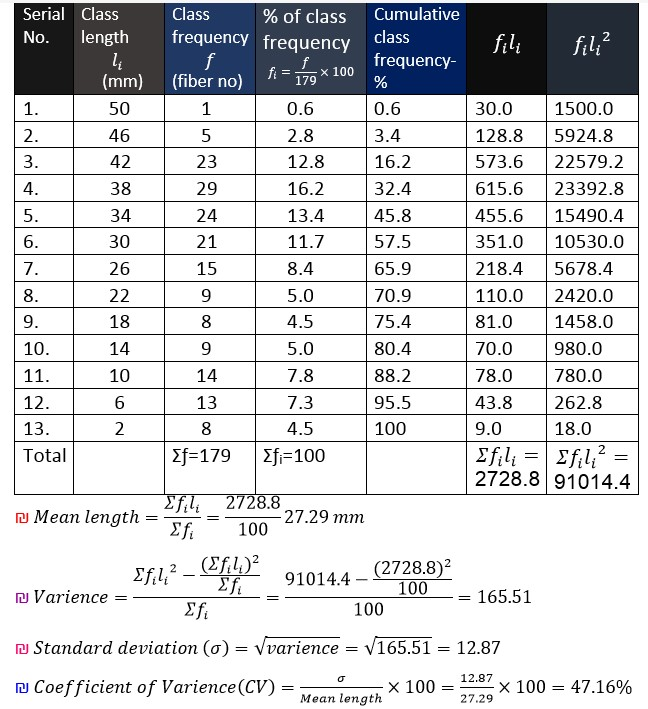Coefficient of fiber length variation (CV %):

The coefficient of variation of fiber length CV % is the ratio of σ divided by the mean length ML. Where σ is the standard deviation of fiber length.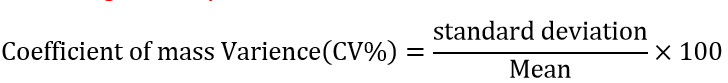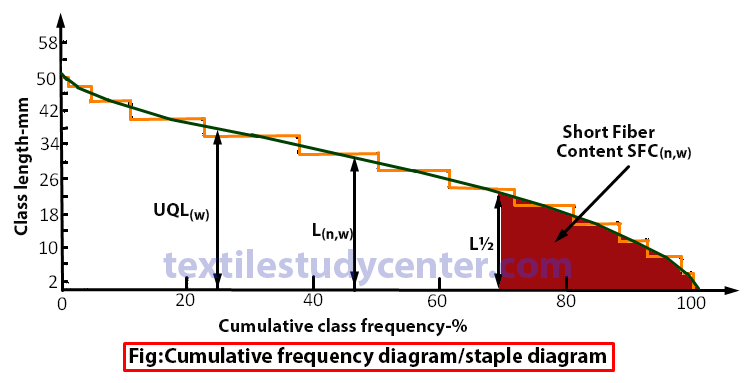Modal Length:

Modal length is the length in a fiber length frequency diagram, which has the highest frequency of occurrence. The modal length for long staple cotton is more than the mean length because of the progressive increase in the skewness of the fiber length distribution with increasing staple length.

Calculation of fiber length (on the basis of number & weight) from staple diagram

a) Mean Length (ML):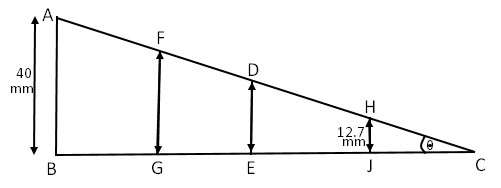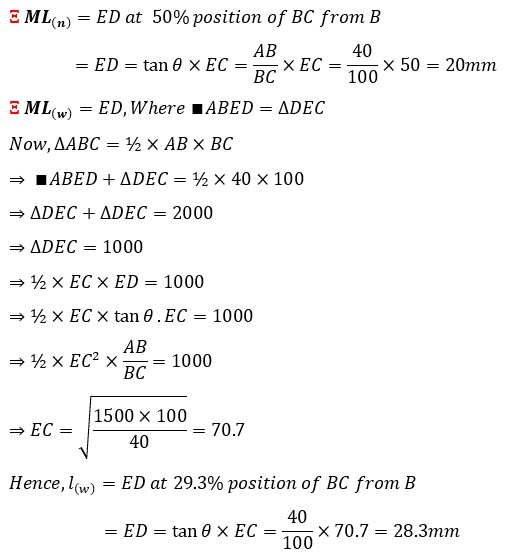b) Upper Quartile Length (UQL):

UQL is the value of length for which 75% of all the observed values are lower, and 25% higher in the fiber sample.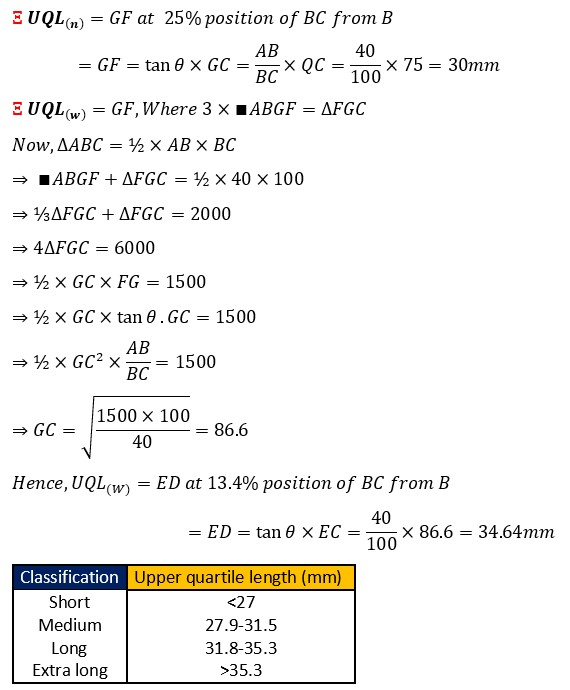c) Short Fiber Content (SFC):

SFC is the percentage by number or weight of fibers less than a specified length, 0.5 inches (12.7mm) for cotton, typically 25 or 40mm for wool.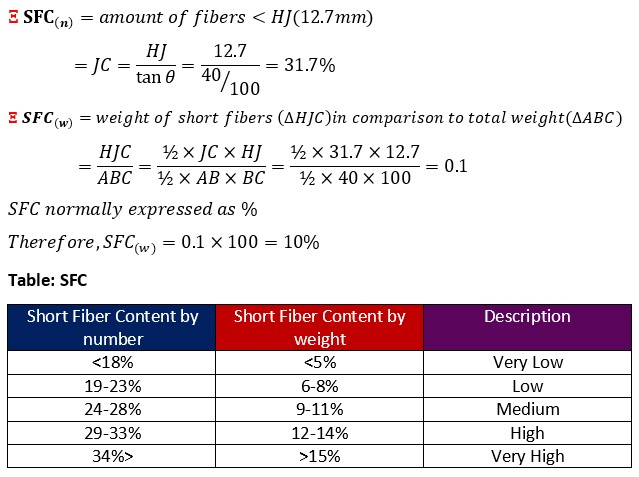(7882)Md Sohanur Rahman Sobuj

Co-founder , Admin & Author at Textile Study Center
Student of Bangladesh University of Textiles (BUTEX) Department: Apparel Engineering+Md Sohanur Rahman Sobuj